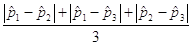## Step 4: Draw inferences beyond the data

Of course, it is possible that there is no difference in the probability of donating among these three treatments, and we just happen to see differences in the sample proportions by random chance. To explore this, we need to first consider what statistic we will use to measure the differences among the three groups. One possibility is the Mean Group Difference, the mean of the three pairwise differences:(g) Calculate the Mean Group Difference statistic for these data (show your work). (You can verify your calculation in the applet.)

(h) What types of values (e.g., large, small, positive, negative) for this Mean Group Difference statistic will provide evidence against the null hypothesis? Explain briefly.